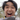# What is __name__ in Python?

June 29, 2020

You’ve most likely seen the following piece of code at the bottom of a Python script:

``if __name__ == ‘__main__’:``

You’ve most likely seen the following piece of code at the bottom of a script:

``````if __name__ == '__main__':
# some code here``````

While this line of code may seem mundane, it’s actually quite powerful! It allows you to run python code as standalone scripts or import them as modules.

## How does it work?

The `__name__` variable is a special “dunder” or magic variables in Python. Dunder stands for “double underscore”, hence the two underscores on both sides.

Dunder variables hold special meaning in Python. The value of the `__name__` dunder variable depends on how you execute the script.

If you run your script from the command line, the `__name__` has the value `__main__`. If you import it, it will contain the name of the script.

Let’s see how this works through code.

## A simple example

Suppose you’re making a simple greeter module. You want it to behave as follows:

• When it’s run as a standalone script, it asks the name of the user and prints “Hi, {user}!“. It repeats this until the program exits.
• When it’s imported, it exposes a `greet` function that can create a greeting given a name

Seems simple enough! Let’s code it.

``````# greeter.py

function greet(name):
print(f'Hi, {name}!')

print('__name__ is:', __name__)

if __name__ == '__main__':
while True:
name = input('> ')

if name == 'q':
break

greeter(name)``````

When you execute this script, you’ll see the following output:

``````__name__ is: __main__

The magic happens before the code runs. Python assigns the value `__main__` to `__name__` since the script is ran standalone.

## Importing our greeter function

What happens then if you import this script is a module from another script?

``````# client.py

from greeter import greet

names = ['Jeff', 'Annie', 'Britta']

for name in names:
greet(name)``````

When you run this, you should see the following output:

``````__name__ is: greeter
Hi, Jeff!
Hi, Annie!
Hi, Britta!``````

Since we imported `greeter` as a module, Python assigned the name of the module, i.e. greeter, as the value of `__name__`. Due to this, the code block inside `__main__` never gets executed.

## Conclusion

Congratulations! You made Python script that you can execute standalone or as a module. All you had to do was to include the following `if` statement:

``````if __name__ == '__main__':
# some code here``````

You can find the sample code used in this article right here.

As a recap, this works because when you run a Python script, the `__name__` dunder variable is:

• `__main__` if you run the script from the command line
• The name of the module if you import it from another script

I hope this short tutorial was helpful!Hi! I'm Adrian. I'm a software engineer, and I work hard to provide helpful and highly intuitive content for free. If you like what you read, please consider following me on Twitter. Hope to see you again next time!